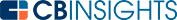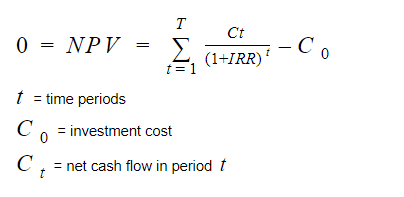# What is an Internal Rate of Return?Internal rate of return (IRR) estimates a potential investment’s profitability. It measures an investment’s expected yearly rate of growth.

## How is an internal rate of return calculated?

Because the internal rate of return is a prediction of a future outcome, it’s necessary to estimate some of its variables. It’s a complex formula that can be calculated by trial-and-error methods. Most commonly, IRR is calculated using a software tool like a financial calculator or Microsoft Excel.

IRR is the annual return that causes net present value (NPV) to equal 0. To determine IRR, use the following formula and set NPV equal to 0.## Who uses the internal rate of return formula?

Several types of investors and companies use internal rate of return, commonly in the real estate, venture capital, and private equity industries. Company leaders use the IRR formula to determine which projects to undertake. The higher the internal rate of return, the greater the likelihood of a project’s financial success.

## What is a good internal rate of return?

An internal rate of return is favorable if it’s greater than a company’s cost of capital or hurdle rate. The hurdle rate is the minimum return investors expect to receive on an investment.

## What does the internal rate of return leave out?

An internal rate of return is a percentage, and it fails to provide hard dollar amounts. For example, a company board of directors wants to decide whether to go with Project A or Project B. The IRR for Project A is 15%, and the IRR for Project B is 20%.

While it’s tempting to assume Project B is favorable based on this data, the dollar amount is missing. Is Project B’s IRR 15% of \$2,000 or 15% of \$200,000? Project B’s IRR is higher, but it could be a larger percentage of a smaller dollar amount compared to Project A.

Internal rate of return also assumes a project’s positive cash flows are reinvested at the IRR. In reality, a project’s positive cash flows are typically reinvested at the company’s cost of capital. To take this into account, investors should use the modified internal rate of return (MIRR) formula shown below.Like the IRR formula, a financial calculator or Microsoft Excel is typically used to calculate MIRR.

An internal rate of return is a measurement used by different investors to estimate a potential investment’s growth rate.

It’s a complicated formula that is typically calculated using financial software instead of manually. A higher internal rate of return is more favorable, and a rate greater than a company’s hurdle rate is desirable.

An internal rate of return measurement is not perfect. It leaves out dollar amounts and makes assumptions about the reinvestment of a company’s cash flows. The modified internal rate of return formula provides a more accurate percentage.

You may also like# www.cbinsights.com